# Multiples of 2

Multiples of 2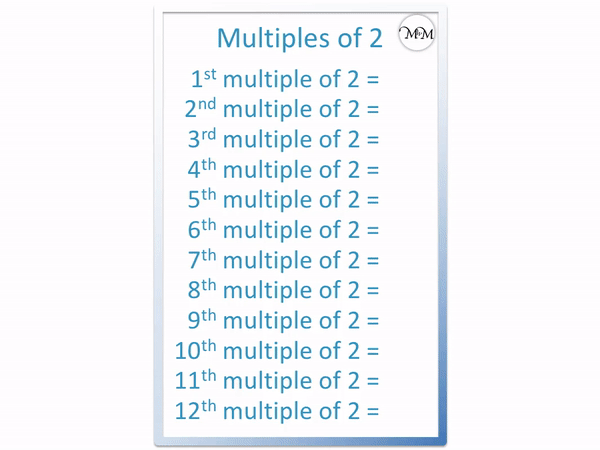• The multiples of 2 are numbers that can be divided by two exactly, leaving no remainder.
• The answers to the two times table are the first few multiples of 2.
• 1 × 2 = 2 and so, the first multiple of two is 2.
• 5 × 2 = 10 and so the fifth multiple of two is 10.
• Multiples of 2 are all even and always end in the digits of 2, 4, 6, 8 or 0.

A multiple of 2 is any number that can be divided by two exactly, leaving no remainder.

The two times table makes up the first few multiples of 2.Click on the multiples of 2 flashcards below to memorise the two times table.

×
×

=#### Multiples of 2 Interactive Question Generator

Multiples of 2: Interactive Questions

# What are the Multiples of 2

Multiples of 2 are numbers that can be divided exactly by 2, leaving no remainder.

The answers to the two times table make up the first few multiples of 2.

The first multiples of 2 are: 2, 4, 6, 8, 10, 12, 14, 16, 18, 20, 22, 24.

The chart below shows the full list of multiples of 2 to 100.There is no final multiple of 2 because the two times table keeps on going.

The two times table is one of the first times tables to learn because the numbers are smaller and more familiar.

Here is a chart showing the two times table.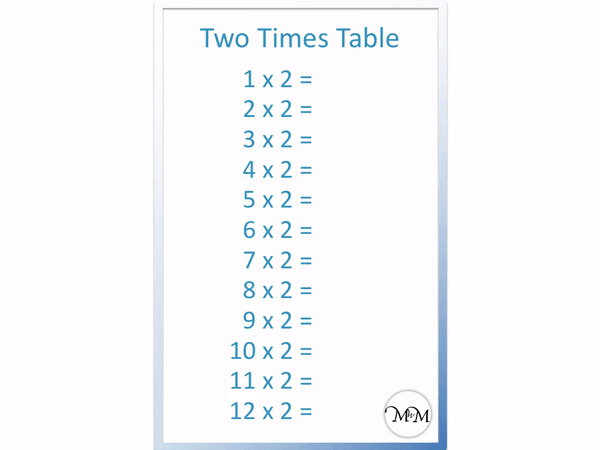The two times table is as follows:

• 1 × 2 = 2
• 2 × 2 = 4
• 3 × 2 = 6
• 4 × 2 = 8
• 5 × 2 = 10
• 6 × 2 = 12
• 7 ×2 = 14
• 8 × 2 = 16
• 9 ×2 = 18
• 10 × 2 = 20
• <11 × 2 = 22
• 12 × 2 = 24

We usually learn the first twelve multiples when learning a times table.The answers to the two times tables are what we call the multiples of 2.To find any particular multiple of two, multiply that given number by two.

For example:

• 1 × 2 = 2 and so the 1st multiple of two is 2.
• 2 × 2 = 4 and so the 2nd multiple of two is 4
• 3 × 2 = 6 and so the 3rd multiple of two is 6
• 4 × 2 = 8 and so the 4th multiple of two is 8
• 5 × 2 = 10 and so the 5th multiple of two is 10
• 6 × 2 = 12 and so the 6th multiple of two is 12
• 7 ×2 = 14 and so the 7th multiple of two is 14
• 8 × 2 = 16 and so the 8th multiple of two is 16
• 9 × 2 = 18 and so the 9th multiple of two is 18
• 10 × 2 = 20 and so the 10th multiple of two is 20
• <11 × 2 = 22 and so the 11th multiple of two is 22
• 12 × 2 = 24 and so the 12th multiple of two is 24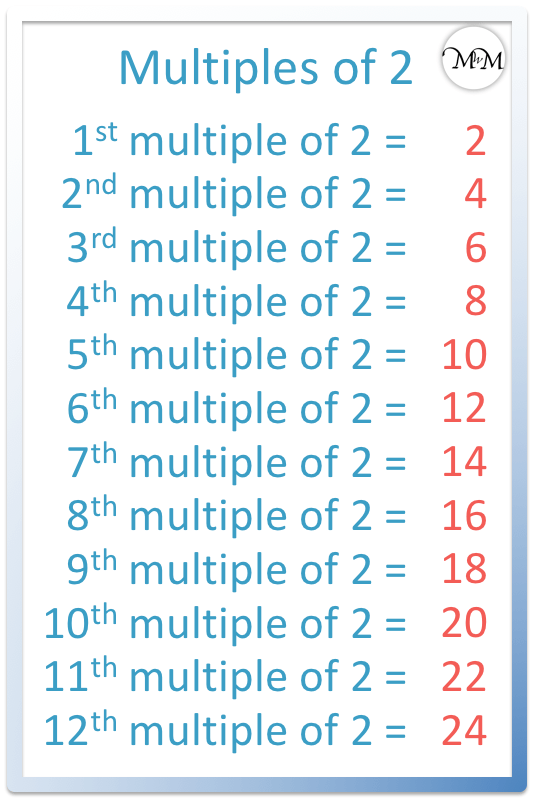When teaching the two times table it is important to recognise patterns in the multiples of 2.

It is helpful to teach larger multiples of 2 using a number grid.

We will use this number grid to 100 to list all multiples of 2 up to 100.The list multiples of 2 up to 100 are:

2, 4, 6, 8, 10, 12, 14, 16, 18, 20, 22, 24, 26, 28, 30, 32, 34, 36, 38, 40, 42, 44, 46, 48, 50, 52, 54, 56, 58, 60, 62, 64, 66, 68, 70, 72, 74, 76, 78, 80, 82, 84, 86, 88, 90, 92, 94, 96, 98, 100.

All of the multiples of 2 can be divided by 2 with no remainder. We can see that the multiples of two always fall within the same columns of the number grid.

We can recognise a pattern in the multiples of 2.

All multiples of 2 end in the digits: 2, 4, 6, 8, 0 and this pattern repeats in this order.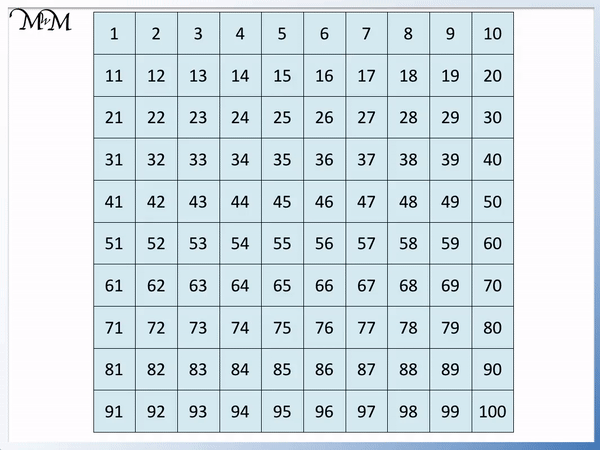The easiest way to remember the two times table is two repeat the pattern of 2, 4, 6, 8 and 0 and increase the tens digit in front each time.

For example we first have 2, 4, 6, 8 and 10.

On the next row of the number grid we have these digits with a ‘1’ digit in front.

We have 12, 14, 16, 18, 20.

We then have a ‘2’ digit in front of the next row.

22, 24, 26, 28 and then 30.

Once you have taught this pattern, the multiples of two continue like this forever.

We can see this pattern in the number grid below, with the final digit in each column being the same but the tens digit increasing by one as we move down each row.Even is a word to describe numbers in the two times table.

We say that all multiples of 2 are even.Now try our lesson on Multiples of 4 where we learn the numbers in the 4 times table.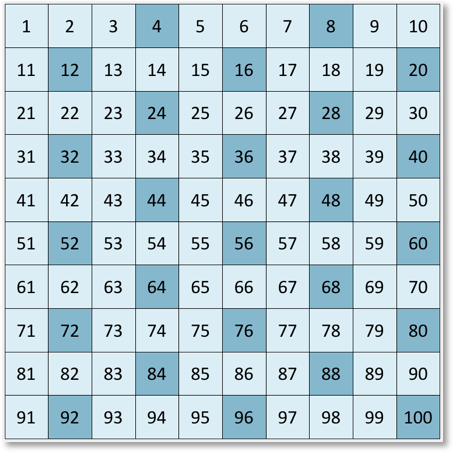error: Content is protected !!